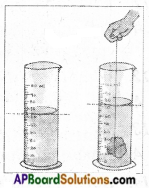AP State Syllabus AP Board 6th Class Science Solutions Chapter 7 Let us Measure Textbook Questions and Answers.

## AP State Syllabus 6th Class Science Solutions 7th Lesson Let us Measure

### 6th Class Science 7th Lesson Let us Measure Textbook Questions and Answers

Fill in the Blanks.
1. Millimetre is a unit for measuring ——–.
A. length
2. For measuring long distances we use ——– as a unit.
A. kilometre
3. The measure of the extent of a plane surface occupied by an object is called ——–.
A. area1. The smallest distance that you can measure with a centimetre scale is [ B ]
A) Centimetre
B) Millimetre
C) Metre
D) Micro Metre
B) Millimetre

2. Volume of solids is measured in [ C ]
A) Metres
B) Square Metres
C) Cubic Metres
D) Centimetres
C) Cubic Metres

3. this is used to measure the area of a irregular plane surface [ B ]
B) Graph paper
C) Measuring Cylinder
D) Scale
B) Graph paper

1. A school hall measures 20 m in length and 15 m in breadth. Find its area.
The length of hall (L) = 20 m
The breadth of hall (B) = 15 m
The area of hall = l × b
= 20 m × 15 m = 300 m2 = 300 sq. m.

2. Ramu’s father had a rectangular plot of length 60 ft. and breadth 50 ft. He built a house occupyinglength 40 ft. ofthepoltand breadth 40 ft. and in the remaining area he planted a garden. Can you help Ramu to find out the area of his garden?
The Area of Rectangular plot A = L1 × B1 Here L1 = 60ft, B1 = 50ft
A1 = L1 × B1 = 60ft × 50ft = 3000 square feet.
The Area of house A2 = L2 × B2 Here L2 = 40ft, B2 = 40ft A2 = L2 × B2 = 40ft × 40ft = 1600 square feet
The remaining area A3 = A1 – A2 = 3000 – 1600 = 1400s feet A3 = 14 × B2 = 40ft × 40ft = 1600 square feet
The remaining area A3 = A1 – A2 = 3000 – 1600 = 1400s feet A3 = 1400 Square feet.
So the area planned for garden = 1400 square feet.3. What questions do you ask a mason to know how he is taking measurements while constructing the building?

• What is the instrument used to measure the length and breadth of an area?
• How many bricks we need to build a wall?
• How much cement and sand we have to mix to prepare concrete?
• What is the instrument used to break the bricks?

4. How can you measure the thickness of a metal wire? Explain?

• Take a metal wire and a pencil.
• Coil the metal wire around the pencil.
• Now measure the length of metal wire by using a scale.
• Divide this with coils of metal wire around pencil. Then we get the thickness of a metal wire.

5. How can you measure the length of a banana? Explain?

• The length of a banana can measure by a common scale.
• Take a 30 cm long scale.
• Keep the banana on a scale, and note the two ends on scale.
• Remove banana and count the centimeters.

6. How can you measure the area of your palm using graph paper? Explain.

• Take a graph paper and place your hand on it.
• Draw an outline of your palm by using a pencil.
• Remove your hand and you can find an outline of your hand on the graph paper.
• Now count the number of complete squares inside the boundary of palm:
• Then count the squares which are half or greater than half as a full square.
• The squares which are less than half are to be neglected on graph papers.
• If there are ‘n’ squares in the boundary, then the area of palm becomes ‘n’ cm2
• This process gives us the value of area of a palm.

7. A carpenter who makes wooden furniture, needs accuracy in measurements. Do you ever notice how he measures? How would you appreciate him?

• The carpenters takes measurements with a metal tape.
• He takes measurements very accurately and nearest to a millimeters.
• As he is a practical worker he has to take correct measurment. If any wrong measure-ment is taken it will affect the furniture that he is making.
• So the carpenters put his mind and concentration on the measurements as his quality of work depends on these measurements.
• Otherwise it will be loss of time and money.

8. The distance between numbers in a clock is accurately the same. List out the things that you observe in your surroundings with accurate distance between them.

• The distance between the kilometer stones on the road is same.
• The radius between front and back wheels of cycles, scooters is exactly same.
• The distance between wings of fan is also same etc.Activities and Projects

6th Class Science Textbook Page No. 82

Question 1.
Measure the volume of “Kalakanda ” (sugar crystal) and piece of “Patika ” (alum). Record your measurements.

• The volume of Kalakanda and Patika are measured by taking kerosene in the measuring cylinder.
• The measurements are recorded in the table.
 Name of the Student Volume of Kalakanda Volume of Patika RAMESH 30 CC 40 CC VENKAT 28.5 CC 42.1 CC GEETHA 27.6 CC 41.8 CC SHAHEENA 25.1 CC 42.7 CC
• All the volumes of Kalakanda measured by students are not equal.
• All the volumes of Patika measured by the students are not equal.
There is parallax error in observing the readings by the students. So there is small variation in their readings.

Question 2.
Make a visit to panchayat office and collect information how VRO measures areas of agricultural lands in your village. Prepare a questionnaire for this.

• Areas of agricultural lands are not measured with the measuring instruments known to us.
• As the measurements involves large distance, VRO uses chains to measure.

QUESTIONNAIRE:

• Why do not you use tape for measurement?
• What is the advantage of using a chain?
•  Do you get accurate measurement with chain?
• Can we use meter scale to measure the length of a place?
• How many links we need to measure 1 acre of land?Question 3.
Collect any invitation card with envelope. Find out the difference between measurements of card and cover. Write down the process that you follow.

• 1. Measurements of covers:
Length of cover L1 = 25 cm; Breadth of cover B1 = 20 cm
• 2. Measurements of card:
Length of card L2 = 23 cm; Breadth of card B2 = 17 cm
• The lengths and breadths of cover and card are measured with the scale.
• The card suitable fits in the cover.

Question 4.
Try to imagine the area of CD, sim card, the mobile phone then find out the area of the above by using graph paper. Compare the values of your guess with graph paper measurement. Which thing is closely related to your guess?
Student Activity.

6th Class Science 7th Lesson Let us Measure Activities

Activity – 1

Measuring Lengths. (Page No. 71)

1. Measure the length of one side of a table using your hand-span (Fig. ).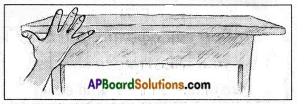Ask your classmates to do the same. Record the length of the table in terms of a number of handspans in the given table:

 S.No. Name of the Student Name of Hand Spans 1. RAMA RAO 6 2. MAHESH 7 3. RAJIYA 8 4. GAYATHRI 8 5. DAVID 9

i) Do all of you get the same number of handspans for the length of the table?
No. I got different number of hand-spans for the length of the table.

ii) Who got more number of hand-spans?
David got more number of hand-spans.

iii) Why is there a difference in number of hand spans, though you measured the same table?

1. Hand spans size is differ from person to person and also from child’s to adults.
2. The students who have little hand spans take more number in measurements.iv) Now find the length of your classroom using your foot-span. Enter your observations in terms of number of foot-spans in the given table:

 S.No. Name of the Student Number of Foot Spans 1. SAI RAM 21 2. YESUBABU 22 3. S1THA 24 4. RIHANA 23 5. PAVAN 26

v) Is the number of foot-spans same when different students measure the length of the same classroom?
No, foot spans are different.

vi) Who got the most number of foot spans? Why?
Pavan got more number of foot spans, because whose foot span are little, so, he got more number of foot spans.

vii) Who got the least number of foot spans? Why?
Sai Ram got least number of foot spans because he has long foot span.

Activity – 2

2. How do you measure the height of your classmate using a meter scale? (Page No. 74)
Make a mark on the wall exactly above his/her head.
Now measure the distance, from the floor to this mark on the wall, with a scale.
Let all other students measure this length in a similar way. Record your observations in your notebook.
i) Study carefully the measurements reported by different students. Do you all have the same readings of measurements?
No, the measurements are different.

ii) If not, what could be the reason for the differences?
Though the measurement was done using a standard scale, results may be close to each other but not exactly equal. The difference in reading is due to some errors in measurement.Activity – 3

3. How do you measure the thickness of coins? (Page No. 76)

• Take about 10 one rupee coins of the same size and place them one upon the other as shown in the figure.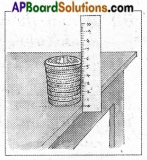• Measure the total thickness with a scale and then divide it by the number of coins to get the thickness of one coin.

Activity – 4

4. How do you find the length of a curved path? (Page No. 76)

• Fix pins at the ends of curved line to be measured as shown in figure.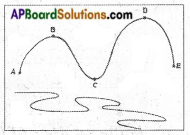• Now tie a knot with cotton thread at the first point ‘A of the pin A and move the cotton thread along with points B, C, D, E etc.
• Care should be taken that the thread is neither too tight nor too lose and see that the thread coincides with the curve at each point while moving along the path.
• When the thread reaches the extreme end of the curved path cut it at that point.
• Remove the thread from A and then place it straight along the length of a meter scale and measure its length.
• The length of the thread is the measure of the length of the curved path.Activity – 5

5. Can you decide which is the bigger and which is the smaller sheet by observing them? If not, what method do you adopt to decide the bigger one or smaller one? (Page No. 76)

• Take two sheets of A4 paper and cut them in the shapes of shown in Figure.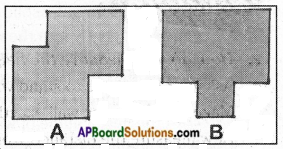• Now take some empty matchboxes of equal size and keep them on the sheet.
• Starting from one corner of the sheet, count how many matchboxes are needed to cover the entire surface of the sheet.
• Similarly repeat the process for the second sheet also and record the findings in your notebooks.
• Which sheet needs more number of matchboxes? Which is bigger in size?
• You may find that one of the sheets needs more number of matchboxes which shows that one sheet is bigger in size than the other.
• Thus, we need to measure the surface of an object to decide whether it is bigger or smaller.
• Area is the measure of the extent of plane surface occupied by an object.
• With this activity, a matchbox is taken as a unit to measure area but it is not a standard unit. We need a standard unit to measure the area.

Activity – 6

Measurement of irregular plane surface: (Page No. 78)

6. How do you find the area of an irregular plane surface (a leaf)?

• Let us find out the area of a surface, say a banana peel or a leaf, which has an irregular shape.
• Place the leaf on graph paper. Mark the boundary of the piece of leaf on the graph paper with a pencil.
• Now remove the leaf to find the outline or boundary of the leaf on graph paper.
• Count the number of complete squares (each of 1 cm2 area) inside the boundary.
• Also count those squares, inside the boundary, which are half or greater than half. Add this to the number of complete squares.
• This total number of squares inside the boundary gives the area of the leaf. If there are ‘n’ squares inside the boundary, the area of the leaf becomes n cm2.
• Neglect those squares, inside the boundary, which are less than half.
• This process will gives us the value of area which is close to the actual area.Activity – 7

7. How do you measure the volume of irregular solids using a measuring cylinder? (Page No. 80)